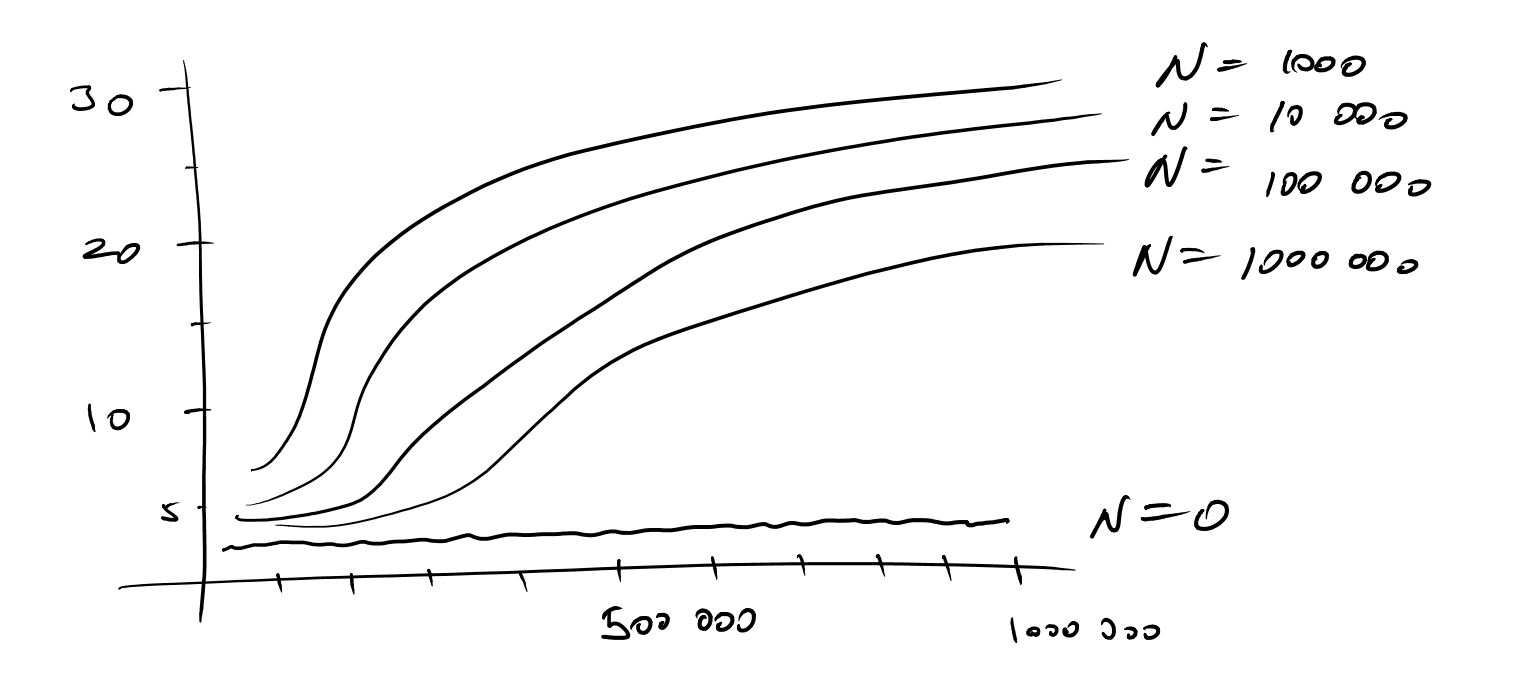Data Structures 1 - NTIN066 - WS 2014/2015

## HW 3

Due date: 15. 12. 2014

### Problem description

Implement lazy binomial heaps. Create a program which reads the test data from the standard input and with each Delete-Min operation it outputs the deleted minimum. The input data are partitioned into runs. Each run constists of a sequence of Insert and Delete-Min operations. The Insert operation starts by the character 'I' in the input. The Delete-Min operation starts with 'D'. The last row of the run is the character 'R'. After each run (at the end of the input) there is a row with the character 'X'. The inserted elements are from the interval [0, n - 1]. After each run the binomial heap is emptied. Each run should be saparated by a newline in the output.

Using unix executable /afs/ms.mff.cuni.cz/u/k/koucky/ds1/pbl3-gen-data which is available in Rotunda laboratory you can generate test data. Test your implementation using those data and for each run measure the overall number of merges during Delete-Min operation. Then measure the count N of Insert operations and the number D of Delete-Min operations. Create a graph (each run is a point):

• The x axis is N - the number of insertions.
• The axis y shows (S-min(S,N))/D.
That is create a graph of the average number of merges per deletion. The graph should have five curves for five test data. Also try to explain the graph.

```I 1
I 3
D
I 2
D
I 0
R
I 2
I 3
I 0
I 1
D
R
X
```

```1
2

0
```

### A possible graph### Generating the input data

\$/afs/ms.mff.cuni.cz/u/k/koucky/ds1/pbl3-gen-data XX D

This will generate data for measuring, remember that XX are the last two number of your student number and D is one of {0,1000,10000,100000,1000000} (the five curves). If D=0, then we perform Delete-Min just after all insertions until the heap is emptied. When D<0, then Delete-Min operations are uniformly positioned among N Insert operations. Each run has at least 1 000 inserts and runs up to 1 000 000 insertions. There are approximately 300 runs. Example:

```pbl3-gen-data 72 0       | my-heap
pbl3-gen-data 72 1000    | my-heap
pbl3-gen-data 72 10000   | my-heap
pbl3-gen-data 72 100000  | my-heap
pbl3-gen-data 72 1000000 | my-heap
```